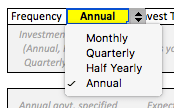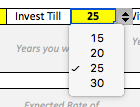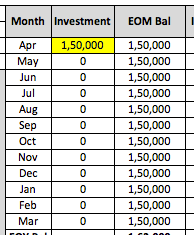(PPF Interest Maturity Calculator – Updated 2023)Are you looking for a PPF Calculator that uses latest PPF interest rates (2023) to calculate the interest earned on PPF investments?

And you also want to know the answer to the big question – what will be PPF maturity amount?

If the answer to above questions is yes, then here is something that you will find useful.

I have created a comprehensive Excel-based PPF calculator that calculates the maturity amount and interest earned depending upon the type of investment you make (fixed or variable) in your PPF account. It also shows you the interest earned every year in the PPF account.

PPF (or Public Provident Fund) needs no introduction.

It is one of the most popular investment-cum-tax saving option among Indian savers.

It’s a tax-deductible investment option that offers reasonable tax-free returns to savers. And it is among the very few risk-free tax saving investment option for us Indian taxpayers (as it provides tax benefits under the Section 80C).

Many people ensure that they utilize the full investment limit of their PPF accounts every year. So naturally, they are concerned about the rate of interest on their PPF accounts and also about estimating the final PPF maturity amount.

I have created this excel based PPF calculator that can be useful if you wish to estimate how much money can be accumulated using your PPF account.

Using this calculator, you will easily find answers to questions like how much you can invest in PPF? How much interest is earned by PPF account? How will the investment grow over the years? The final PPF maturity amount?

And since you can control the investment as well as maturity tenure in the excel sheet, it can be your goto option if you are searching for:

• PPF calculator for 15 years, or
• PPF calculator for 20 years, or
• PPF calculator for 25 years, or even
• PPF calculator for 30 years

All you have to do is to provide few inputs and this PPF excel sheet will tell you everything you need to know about your PPF investments.

Decide your PPF investment frequency (Monthly, Quarterly, Half Yearly, Annual)Decide till when you wish to keep investing in PPF (15, 20, 25 or 30 years)Decide when you finally wish to withdraw money from PPF (end of 15th, 20th, 25th or 30th year)PPF accounts are opened for an initial term of 15 years and once the lock in period of 15 years is over, the subscriber can withdraw the full amount at the time of maturity.

PPF account withdrawal rules allow for partial withdrawals even before the 15-year lock-in is over. But we will ignore that scenario as we are concerned about estimating the maturity amount of an undisturbed and a fully funded PPF account.

Luckily, PPF rules allow that the account can be extended in blocks of 5 years.

PPF Subscribers can choose whether they want to extend with contributions or without contributions. So if you decide, you can continue investing even after the 15th year.

So using the two inputs of your investment period and maturity/withdrawal year in the calculator, you can figure out the final maturity amounts for the following combinations:

• Invest in PPF for 15 years. Withdraw at end of 15th year
• Invest in PPF for 15 years. Withdraw at end of 20th year
• Invest in PPF for 15 years. Withdraw at end of 25th year
• Invest in PPF for 15 years. Withdraw at end of 30th year
• Invest in PPF for 20 years. Withdraw at end of 20th year
• Invest in PPF for 20 years. Withdraw at end of 25th year
• Invest in PPF for 20 years. Withdraw at end of 30th year
• Invest in PPF for 25 years. Withdraw at end of 25th year
• Invest in PPF for 25 years. Withdraw at end of 30th year
• Invest in PPF for 30 years. Withdraw at end of 30th year

Apart from this, the calculator also gives the option of setting the annual investment limits and interest rates (yellow cells) for each year separately.Both the PPF account interest rate and annual investment limits can be varied for each financial year.

This is useful as the government is known to increase the maximum annual limit of PPF deposit every few years.

Currently, the maximum PPF investment limit per year is Rs 1.5 lakh. You can try out scenarios where this annual PPF limit is increased by say Rs 50,000 every 3-4 years.

Note – The calculator assumes that you would invest the full amount as prescribed by the limit. But you can play around with the excel sheet and bypass this restriction yourself.

The PPF interest rates too don’t remain the same forever.

But the rate of interest in PPF account in post offices, banks or anywhere else is the same at a given point of time. The calculator shows it at 8% for all years 1 to 30. In reality, this will not happen.

The current PPF interest rate is 7.1% (in 2023).

But as per current PPF account rules, the government has the option of changing PPF rates every quarter now. For more information, you can have a look at the historical PPF Interest Rates to get an idea about rate fluctuations and changes in PPF rules in the past.

Note – The interest on PPF investments is calculated on a monthly basis. So all your monthly PPF contributions count. However, the total yearly interest is added to the PPF account at the end of the financial year. This should be remembered when doing PPF interest rate calculation or looking at how to calculate PF amount at the end of the year. The calculator provided in this post has embedded PF calculation formula in excel which you can download freely.

Do remember that the interest on PPF is calculated on the minimum balance in the account between the 5th and the last day of each month. So if you invest monthly, then it makes sense to do it before the 5th of every month. Do read invest before 5th Vs after 5th of month in PPF. Or if you need to invest Rs 1.5 lakh in PPF in lumpsum, then invest Rs 1.5 lakh in PPF before 5th April every year to maximize your benefits.

### Realistic PPF Investment Scenario Testing

You can test out various PPF interest rate and investment limit related scenario using this tool.

Using a combination of increasing annual limits and falling rates (rates are expected to go down gradually as the Indian economy matures), you can try out various scenarios yourself. One such hypothetical scenario can be:

• Year 1-5: PPF annual investment limit Rs 1.5 lakh: PPF rate 8.0%
• Year 5-10: PPF annual investment limit Rs 2.0 lakh: PPF rate 7.5%
• Year 10-15: PPF annual investment limit Rs 2.5 lakh: PPF rate 7.0%
• Year 15-20: PPF annual investment limit Rs 3.0 lakh: PPF rate 6.5%
• Year 20-25: PPF annual investment limit Rs 3.5 lakh: PPF rate 6.0%
• Year 25-30: PPF annual investment limit Rs 4 lakh: PPF rate 5.5%

It will be interesting to see how these changes play out and impact the final maturity amount.

So finally,

And to get you going, here are some examples of the calculations of maturity amounts. Don’t miss the 3rd example:

### Example – 1

Annual Investment (in April) – Rs 1.5 lakh lump sumRate of Interest – 8.0%

Investment Period – 15 years

Maturity Amount (at the end of 15th year) ~ Rs 44 lakh

Maturity Amount (at the end of 25th year) ~ Rs 95 lakh

As you can see, this example illustrates how you can use this tool as a PPF Fixed Annual Investment Calculator.

### Example – 2

Monthly Investment – Rs 12,500 per month (Rs 1.5 lakh annually)Rate of Interest – 8.0%

Investment Period – 20 years

Maturity Amount (at the end of 20th year) ~ Rs 71 lakh

Maturity Amount (at the end of 30th year) ~ Rs 1.54 crore

This is an example of using this excel based calculator for estimating the maturity amount when monthly investments/contributions are made in your PPF account.

### Example – 3 (Realistic)

Annual Investment – Rs 1.5 lakh (years 1-5); Rs 2.0 lakh (yrs 5-10); Rs 2.5 lakh (yrs 10-15)

Rate of Interest – 8% (yrs 1-5); 7% (yrs 5-10); 6% (yrs 10-15); 5% (yrs 15-25)

Investment Period – 15 years

Maturity Amount (at the end of 25th year) ~ Rs 85 lakh

As you see, you can create your own unique scenario basis your views / estimates about the future investment limits and rate of interest on PPF accounts.

So go ahead and play with this calculator to calculate PPF Maturity Value for 15 to 30 years with detailed annual timeline that displays month-wise (or other periodicity-wise) contribution and interest calculation every year in line with applicable PPF interest rates.

I hope you find this Excel PPF interest and maturity calculator useful.

Once you use this PPF Calculator, you will know how to calculate interest on your PPF investment and get an idea about the estimated PPF maturity amount.

You can use it as a PPF maturity calculator for 15 years, 20 years, 25 years or even 30 years. You can even run the numbers to find out How to save Rs 1 crore using PPF and become a PPF crorepati.

Using an online or excel based PPF interest calculator to estimate your maturity amount is fine. But if you are investing for long term financial goals, PPF or other debt instruments might not be enough. You need to have more exposure to equity (why?).

So go on… use this PPF calculator excel xlsx xls sheet and see if you are actually using your provident fund investments (PPF or others) to create wealth or not.

PPF calculator is a very easy way to calculate PPF interest and PPF maturity amount.

And PPF is a solid debt-based savings product that can be used to save some serious money. Don’t ignore it and use it judiciously.

1.Amit says:

The primary advantage is that it’s Tax Free. So, if one chooses to withdraw after five years, and he saves 20%on tax, then he has saved an additional 4% more reach year.

So, till 1.5 lakhs tax limit PPF is boss.

2.Rajesh Medampudi says:

SBI ppf only allows for 5 year extension from the 15 yrs that we are to be invested in.. How and where are the 30 years plans coming from in here. can you please guide us in this.

1.Shrikant Mahajan says:

Extension by 5 years each time is allowed in SBI. If any staff is unaware, which happens with many banks, you can write to its customer care. But PPF is no more earning option for tax relief. You can try ELSS, especially the one with closed ended one. NFO of one such scheme, viz. ICICI long term wealth enhancement fund is getting closed on 21 March. So hurry up.

2.Sapna says:

You can have as many 5 yr extensions after the lock-in period of 15 yrs. ==> There is no upper age limit for opening a PPF account. The lock-in, however, remains at 15 years irrespective of the age at which you open the account. On maturity, the account can be extended by blocks of 5 years any number of times.

3.rangarajan says:

Hi. Very good and useful calculator. Many thanks.

4.Ravi Kumar says:

How can I calculate the intrest monthly,if my monthly installments are not fixed and moving up and down every month.

5.Ashlesha says:

Thanks a million for this!

6.sam says:

Dear Sir, I opened PPF account on 04-04-2016 & the same day i deposited Rs. 5000/- Kindly Let me Know that when account will be mature.